# Probability Distributions Discrete Random Variables Outcomes and Events

• Slides: 6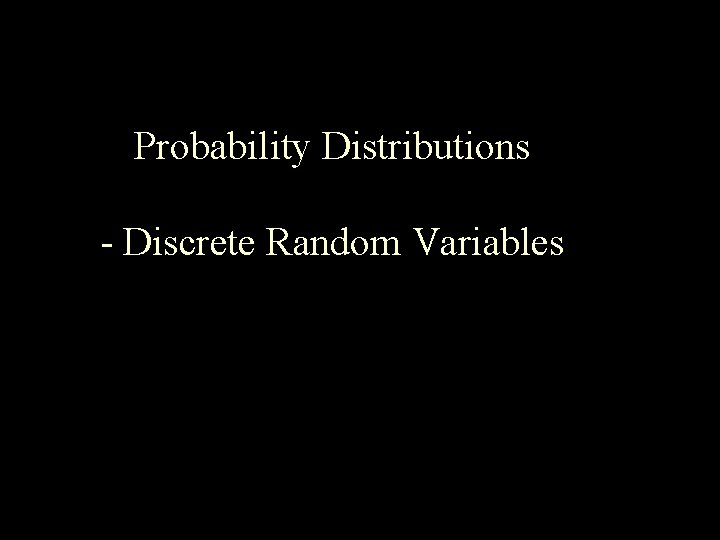Probability Distributions - Discrete Random Variables Outcomes and Events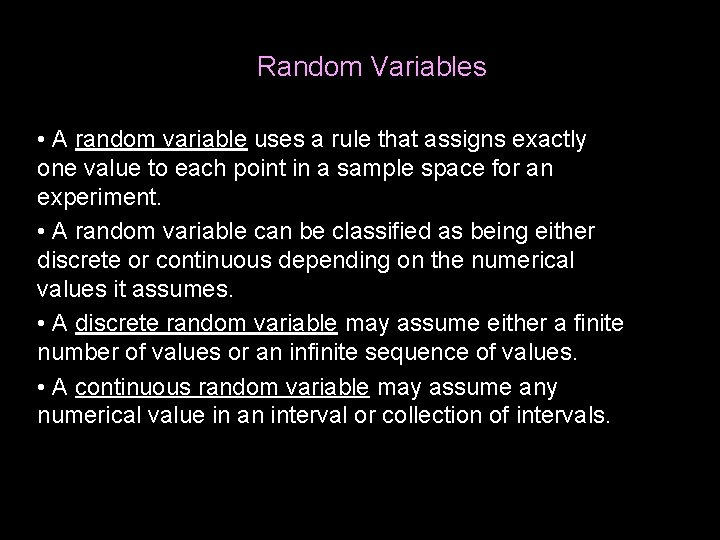Random Variables • A random variable uses a rule that assigns exactly one value to each point in a sample space for an experiment. • A random variable can be classified as being either discrete or continuous depending on the numerical values it assumes. • A discrete random variable may assume either a finite number of values or an infinite sequence of values. • A continuous random variable may assume any numerical value in an interval or collection of intervals.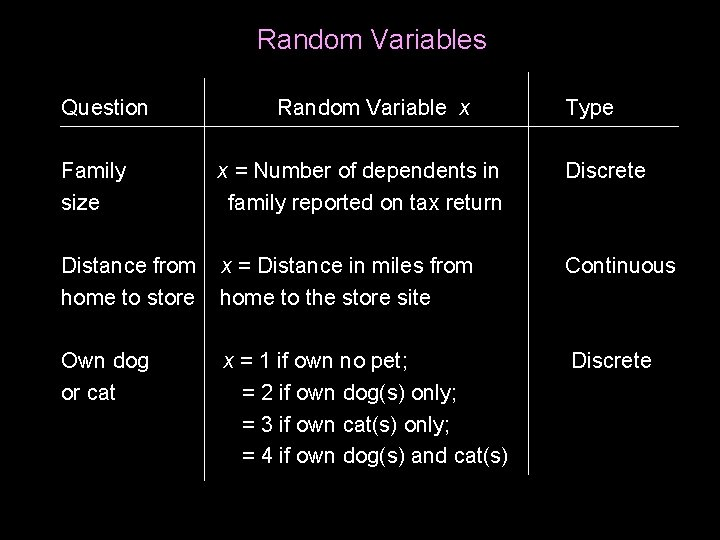Random Variables Question Random Variable x Type Family x = Number of dependents in Discrete size family reported on tax return Distance from x = Distance in miles from Continuous home to store home to the store site Own dog x = 1 if own no pet; or cat = 2 if own dog(s) only; = 3 if own cat(s) only; = 4 if own dog(s) and cat(s) Discrete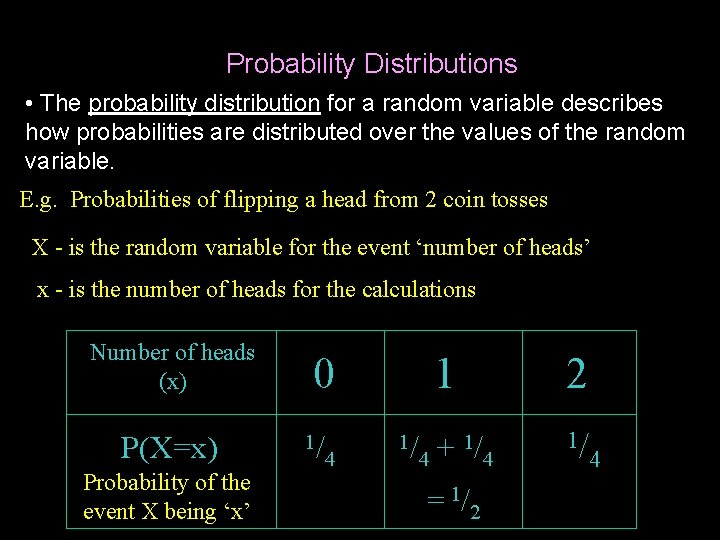Probability Distributions • The probability distribution for a random variable describes how probabilities are distributed over the values of the random variable. E. g. Probabilities of flipping a head from 2 coin tosses X - is the random variable for the event ‘number of heads’ x - is the number of heads for the calculations Number of heads (x) P(X=x) Probability of the event X being ‘x’ 0 1/ 4 1 1/ 1/ + 4 4 = 1/2 2 1/ 4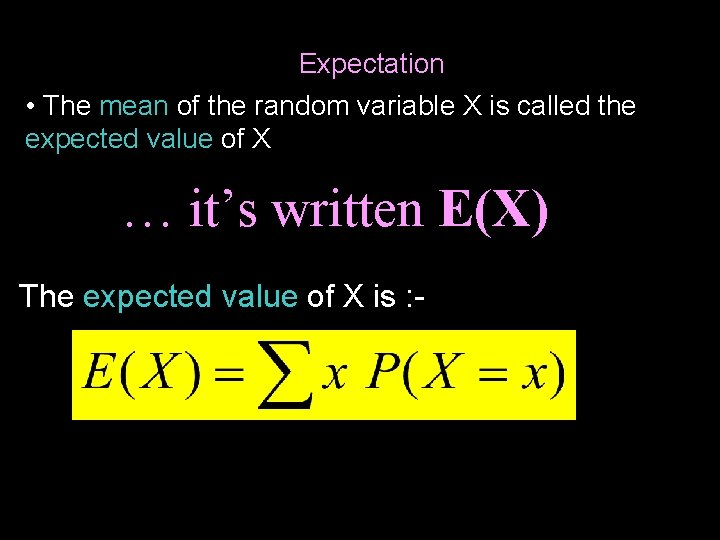Expectation • The mean of the random variable X is called the expected value of X … it’s written E(X) The expected value of X is : -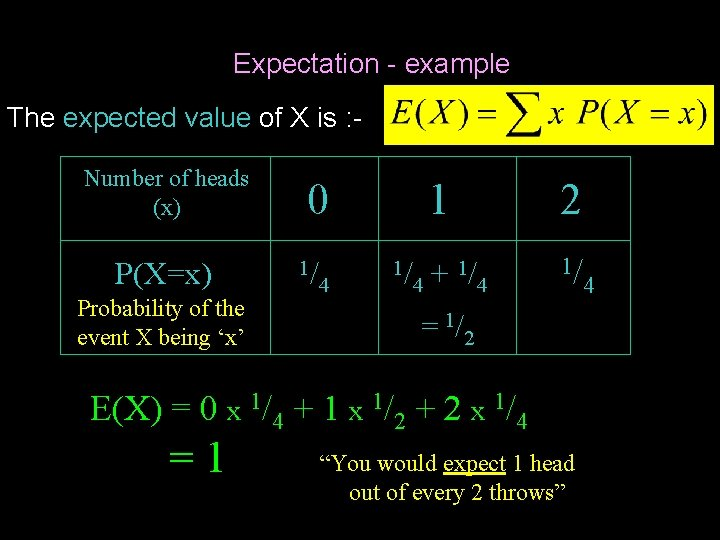Expectation - example The expected value of X is : Number of heads (x) P(X=x) Probability of the event X being ‘x’ 0 1/ 4 1 1/ 1/ + 4 4 2 1/ = 1/2 E(X) = 0 x 1/4 + 1 x 1/2 + 2 x 1/4 =1 “You would expect 1 head out of every 2 throws” 4